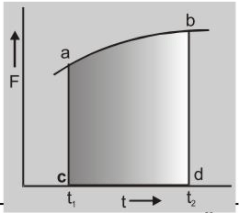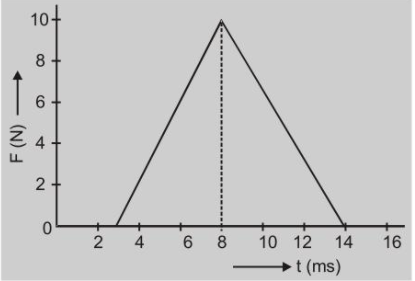Most Affordable JEE | NEET | 8,9,10 Preparation by Kota's Top IITian Doctor Faculties

# Applications of impulse - Impulse, Physics - eSaral`
Hey do you want to learn about the applications of impulse? If so, Then you are at the right place

## Impulse

It is not convenient to measure the varying force of impact. Suppose that $\overrightarrow{\mathrm{F}}$ av is the average force acting during impact and $t$ is the small-time for which the impact lasts.

In such situations, it is found that the quantity Fav. It is very easy to measure.

The quantity $\overrightarrow{\mathrm{F}}_{\text {av }} . \mathrm{t}$ is called impulse.

If a variable force acts on a body or particle for a interval of time $t_{1} \rightarrow t_{2}$

Impules is

$\overrightarrow{\mathrm{I}}=\int_{t_{1}}^{t_{2}} \overrightarrow{\mathrm{F}} \mathrm{dt}=\overrightarrow{\mathrm{p}_{2}}-\overrightarrow{\mathrm{p}_{1}}$

Impules momentum theorem

The integral $\int_{0}^{t} \overrightarrow{\mathrm{F}} \mathrm{dt}$ is measure of the impulse, when the force of impact acts on the body and from equation, we find that it is equal to total change in momentum of the body.

Dimensional formula $\&$ unit. The dimensional formula of impulse is the same as that of momentum i.e. [ML $T^{-1}$ ]. In SI, the unit of impulse is N-s or kg ms $^{-1}$ and in cgs system it is dyne-s or $\mathrm{g} \mathrm{cm} \mathrm{s}^{-1}$

## Applications of Concept of Impulse :

(a) Bogies of a train are provided with buffers: The buffers increase the time interval of jerks during shunting and hence reduce the force with which the bogies pull each other. $(\because \mathrm{F} \Delta \mathrm{t}=\mathrm{constant})$

(b) A cricket player draws his hands back while catching a ball: The drawing back of hands increases time interval and hence reduces force with which the ball hits the hand.

(c) A person jumping on a hard cement floor receives more injuries than a person jumping on a muddy or sandy road: Because on the hard cemented floor the feet of the man immediately comes to rest and the time is small, therefore, the force experienced by the man is large while on the sandy soil the feet embedes into the soil, thus the time taken for same change in momentum is comparatively increased. Thus the force is decreased.

(d) Cars, buses, trucks, bogies of the train, etc are provided with a spring system to avoid severe jerks. Due to the spring system, the time interval of the jerks increases. As the rate of change of momentum will be smaller, comparatively lesser force acts on the passengers during the jerks.

(e) China wares are wrapped in straw or paper before packing. China wares are wrapped in straw or paper so that when they receive jerks during transportation, the time of impact may be more. As such, only a small force can act on the china wares during jerks and there is no fear of damage to them.

(f) If a graph is plotted between $\overrightarrow{\mathrm{F}}$ and $\mathrm{t}$ with $\overrightarrow{\mathrm{F}}$ as ordinate (Y-axis)

and $t$ as $(X$ -axis)

Impulse = Area enclosed by F-t curve and time axis for specified duration.

For example impulse between time $t_{1}$ and $t_{2}=$ area abdc.Newton's Laws of Motion

To watch Free Learning Videos on physics by Saransh Gupta sir Install the eSaral App.

Ex. A hammer weighing $2.5 \mathrm{kg}$ moving with a speed of $1 \mathrm{m} / \mathrm{s}$ strikes the head of a nail driving it $10 \mathrm{cm}$ into the wall. What is the acceleration during impact and the impulse imparted to wall.

Sol. The initial velocity of nail is same as that of hammer i.e. $\mathrm{u}=1 \mathrm{m} / \mathrm{s}, \mathrm{v}=0, \mathrm{s}=10 \mathrm{cm}=0.10 \mathrm{m}$

$$\begin{array}{l}\therefore \quad v^{2}=u^{2}+2 a s \text { gives } \\0=1^{2}+2 a \times 0.1\end{array}$$

This gives $a=-\frac{1}{0.2}=-5 \mathrm{m} / \mathrm{s}^{2}$

i.e. Retardation $\mathrm{a}=5 \mathrm{m} / \mathrm{s}^{2}$

Impulse imparted to wall = - change in momentum of hammer

$$=-\{\mathrm{M}(\mathrm{v}-\mathrm{u})\}=-\{0.5(0-1)\}=+2.5 \mathrm{N}-\mathrm{s}$$

Ex. Magnitude of the force in newton acting on a body varies with time $t$ (in mili-second) as shown in fig. below. Calculate magnitude of total impulse of the force.

Sol. The impulse

\begin{aligned} \mathrm{I}=\int \mathrm{F} \mathrm{dt} &=\frac{1}{2}(0.014 \mathrm{s}-0.003 \mathrm{s})(10 \mathrm{N}) \\ &=0.055 \mathrm{N} \cdot \mathrm{s}=0.055 \mathrm{kg} \cdot \mathrm{m} / \mathrm{s} \end{aligned}

Area of the graph gives the magnitude of the Impulse.

Ex. $\quad$ A force exert an impulse I on a body changing its speed from u to v. The force and object's motion are along the same line. Show that the work done by the force is $\frac{\mathrm{I}(\mathrm{u}+\mathrm{v})}{2}$.Sol. According to work-energy theorem we have

$$W=\Delta K=\frac{1}{2} m v^{2}-\frac{1}{2} m u^{2} \quad \text { or } \quad W=\frac{1}{2} m(v-u)(v+u)$$

But according to impulse-momentum theorem

$$\mathrm{I}=\mathrm{m}(\mathrm{v}-\mathrm{u})$$

So, eliminating $\mathrm{m}$ between $\mathrm{eq}^{\mathrm{n}} .(1)$ and (2)

$$W=\frac{1}{2} \frac{I}{(v-u)}(v-u)(v+u)=\frac{I(v+u)}{2} \quad \text { This is the required result. }$$

Ex. A cricket ball of mass $150 \mathrm{g}$ is moving with a velocity of $12 \mathrm{m} / \mathrm{s}$ and is hit by a bat so that the ball is turned back with a velocity of $20 \mathrm{m} / \mathrm{s}$. If the duration of contact between the ball and bat is $0.01 \mathrm{s},$ find ther impulse and the average force exerted on the ball by the bat.

Sol. According to given problem change in momentum of the ball

$$\Delta p=p_{f}-p_{i}=m(v-u)=150 \times 10^{-3}[20-(-12)]$$

So by impulse-momentum theorem $I=\Delta p=4.8 \mathrm{N}-\mathrm{s}$

And by time averaged definition of force in case of impulse $\mathrm{F}_{\mathrm{av}}=\frac{\mathrm{I}}{\Delta \mathrm{t}}=\frac{\Delta \mathrm{p}}{\Delta \mathrm{t}}=\frac{4.80}{0.01}=480 \mathrm{N}$